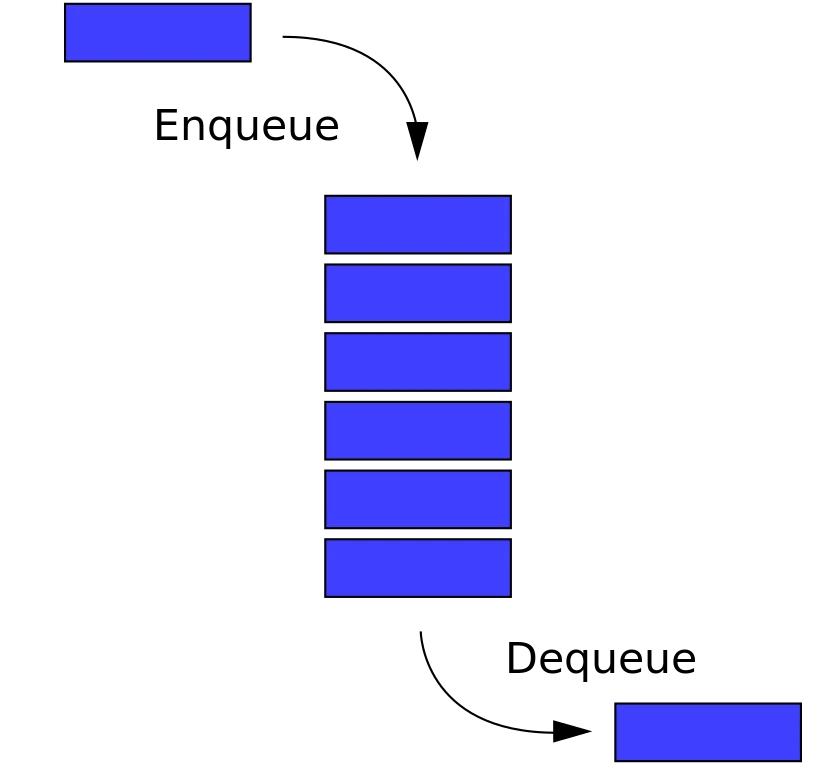# Programming - C Queues using Linked ListsHello, its me again. I was away for the weekend and didn't manage to find time to upload any post. But, for that reason I will upload 2 posts today. We will talk about Queue Implementation using Linked Lists (next post will be Stacks). I will show you the exact same functions (add, remove, print) that we used with Array Implementation. So, without further do, let's get started.

As we already know, a Queue is a Data Structure that implements the FIFO way of getting information. We define the most Data Structures with structs in C. But, because this time we use Lists we will also need a Node for the List. This Node will contain Data and a pointer to the next Node. So our Node looks like this:

``typedef struct Node{ ``
`` int val;``
`` struct Node *next;``
``}Node;``

Using this Node definition we can now have our Queue front and rear be Node pointers! So, our Queue struct will contain those 2 pointers and maybe even other information like capacity of Queue and so on...
The Code looks like this:

``typedef struct Queue{``
`` Node *front;``
`` Node *rear;``
``}Queue;``

Those 2 pointer will be initialized as NULL, that means the Queue is empty!

The functions will again use a struct variable of type Queue, that will be a pointer (we pass the address) when we add or remove (change the Queue in any way) and be passed as a normal variable when printing (so that we don't lose any information).

For adding an Item we can check if Queue is full, when having a capacity variable.

Afterwards, we create a new Node using the Code we used in Linked Lists like this:

``Node *nn;``
``// create new node``
``nn = (Node*)malloc(sizeof(Node));``
``nn->val = val;``
``nn->next = NULL;``

A special Case is when the Queue is empty, where we set front and rear equal to our new Node. The basic Case simpy adds the new Item at the end using a Current Node Pointer and setting the rear to the new Node.

So, our Code looks like this:

``void enqueue(Queue *Q, int val){``
`` Node *cur, *nn;``
`` // create new node``
`` nn = (Node*)malloc(sizeof(Node));``
`` nn->val = val;``
`` nn->next = NULL;``
`` // check if empty``
`` if(Q->front == NULL){``
``     Q->front = Q->rear = nn;``
`` }``
`` // basic case``
`` else{``
``     // set cur to front``
``     cur = Q->front;``
``     // find last Node``
``     while(cur->next != NULL){``
``         cur = cur->next;``
``     }``
``     // insert nn to end``
``     cur->next = nn;``
``     Q->rear = nn;    ``
`` }``
``}``

# Removing (Dequeue):

When removing an Item we have to check if the Queue is empty that means we return -1. Afterwards, we get the value at the front and then we have 2 cases: When this Item was the only one we set both pointers to NULL, else we simply set the front to the front next pointer. Last, we return our value.

So, our Code looks like this:

``int dequeue(Queue *Q){``
`` int val;``
`` // check if empty``
`` if(Q->front == NULL){``
``     return -1;``
`` }``
`` // get value in front``
`` val = Q->front->va>l;``
`` // check if one item inside``
`` if(Q->front == Q->rear){``
``     Q->front = Q->rear = NULL;``
`` }``
`` // basic case``
`` else{``
``     // make front point to next``
``     Q->front = Q->front->>next;``
`` }``
`` //return the value ``
`` return val; ``
``}``

# Printing:

For printing I will call the dequeue function as long as there are Items. We could also use a while loop the way we did it in Linked Lists. But, I think it makes more sense using the dequeue function on a struct variable, so that we don't lose information.

So, this is our Code:

``void print(Queue Q){``
`` int val;``
`` // check if empty``
`` if(Q.front == NULL){``
``     printf("Queue is Empty!\n");``
``     return;``
`` }``
`` // call dequeue as long as there are items``
`` while((val= dequeue(&Q)) != -1){``
``     printf("%d ", val);``
`` }   ``
`` printf("\n");``
``}``

# Code:

Lastly, here a Code that uses those functions so that you know how to set them up. It's almost the same exact Code I used with Arrays.

``int main(){``
`` // initialize Queue``
`` Queue Q;``
`` Q.front = Q.rear = NULL;``
`` print(Q);``
`` // add 5 to queue``
`` enqueue(&Q, 5);``
`` print(Q);``
`` // add 10 to queue``
`` enqueue(&Q, 10);``
`` print(Q);``
`` // remove from queue``
`` int val;``
`` val = dequeue(&Q);``
`` if(val == -1){``
``     printf("Queue is Empty!\n");``
`` }``
`` else{``
``     printf("%d\n", val);``
`` }``
`` print(Q);       ``
``}``

Thanks for reading. Hope you enjoyed it! :)

H2
H3
H4
3 columns
2 columns
1 column
Join the conversation now